Related Articles
How to plot an angle in Python using Matplotlib ?
• Last Updated : 04 Feb, 2021

In this article, we will learn how to plot an angle in Python. As we know that to draw an angle, there must be two intersecting lines so that angle can be made between those two lines at an intersecting point. In this, we plot an angle on two Intersecting Straight lines. So, lets first discuss some concepts :

• NumPy is a general-purpose array-processing package. It provides a high-performance multidimensional array object and tools for working with these arrays.
• Matplotlib is an amazing visualization library in Python for 2D plots of arrays. Matplotlib is a multi-platform data visualization library built on NumPy arrays and designed to work with the broader SciPy stack. It was introduced by John Hunter in the year 2002.

In this matplotlib is used to plot angle graphically and it fits best with Numpy whereas NumPy is numerical Python which is used to perform Advance Mathematics.

### Steps Needed

1. Plot two Intersecting lines.
2. Find the point of Intersection marked with a color.
3. Plot a circle in such a way that the point of Intersection of two lines is the same as Center of Circle.
4. Mark it as a point where the circle will intersect with straight lines and plot that two points where we find the angle between them.
5. Calculate the angle and plot the angle.

### Step-by-Step Implementation

1. Plot two Intersecting lines

• In this, the first two lines of Code shows that matplotlib and NumPy  Framework of Python is imported, from where we will use inbuilt functions in further code.
• After that, the slope and intercept are taken in order to plot two Straight lines. After that line-space ( l ) returns number spaces evenly with respect to the interval.
• After that plt.figure() is used to create the area where we plot the angle and it’s dimension is given in the code.
• After that In order to plot the Straight lines, we have to define the axis. Here : X-axis : 0-6 and Y-axis : 0-6
• The title is given to the figure box using plt.title().

After that two lines are plotted as shown in the output below:

## Python3

 `# import packages``import` `matplotlib.pyplot as plt``import` `numpy as np` `# slope  and intercepts``a1, b1 ``=` `(``1``/``4``), ``1.0``a2, b2 ``=` `(``3``/``4``), ``0.0` `# The numpy.linspace() function returns``# number spaces evenly w.r.t interval``l ``=` `np.linspace(``-``6``, ``6``, ``100``)` `# use to create new figure``plt.figure(figsize``=``(``8``, ``8``))` `# plotting``plt.xlim(``0``, ``6``)``plt.ylim(``0``, ``6``)``plt.title(``'Plot an angle using Python'``)``plt.plot(l, l``*``a1``+``b1)``plt.plot(l, l``*``a2``+``b2)``plt.show()`

Output :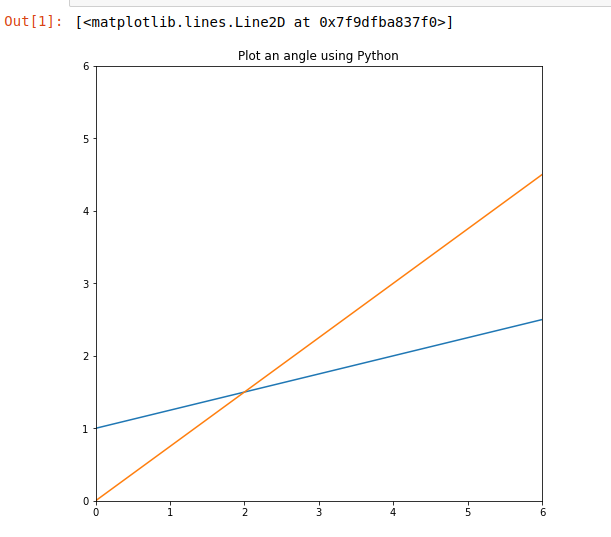2. Find the point of Intersection  and marked with a color

Here x0,y0 denote the intersecting point of two Straight lines. The plotted two Straight lines are written as :

```y1 = a1*x + b1
y2 = a2*x + b2.```

On solving the above equations we get,

```x0 = (b2-b1) / (a1-a2)   -(i)
y0 =a1*x0 + b1             -(ii)```

From above equation (i) and (ii) we will get the point of intersection of Two Straight lines and after that, the color =’midnightblue’ is assigned to the point of intersection using plot.scatter() function.

## Python3

 `# import packages``import` `matplotlib.pyplot as plt``import` `numpy as np` `# slope  and intercepts``a1, b1 ``=` `(``1``/``4``), ``1.0``a2, b2 ``=` `(``3``/``4``), ``0.0` `# The numpy.linspace() function returns``# number spaces evenly w.r.t interval``l ``=` `np.linspace(``-``6``, ``6``, ``100``)` `# use to create new figure``plt.figure(figsize``=``(``8``, ``8``))` `# plotting``plt.xlim(``0``, ``6``)``plt.ylim(``0``, ``6``)``plt.title(``'Plot an angle using Python'``)``plt.plot(l, l``*``a1``+``b1)``plt.plot(l, l``*``a2``+``b2)` `# intersection point``x0 ``=` `(b2``-``b1)``/``(a1``-``a2)``y0 ``=` `a1``*``x0 ``+` `b1``plt.scatter(x0, y0, color``=``'midnightblue'``)`

Output: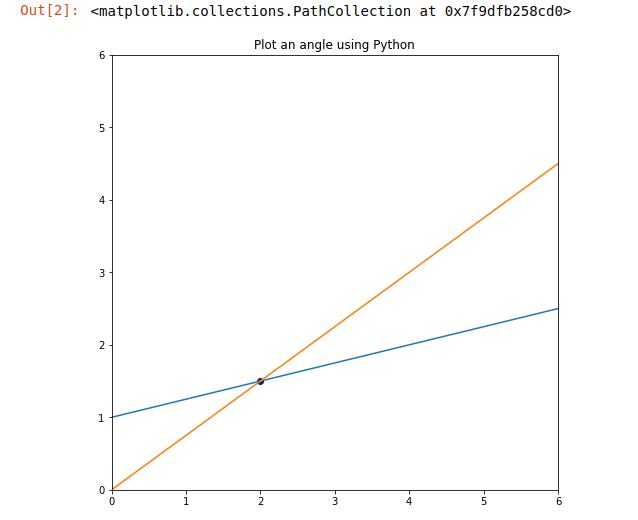3. Plot a circle in such a way that the point of intersection of two lines is the same as the Center of Circle

Here we plot a circle using the parametric equation of a Circle. The parametric equation of a circle is :

```x1= r*cos(theta)
x2=r*sin(theta)```

If we want that the circle is not in the origin then we use :

```x1= r*cos(theta) + h
x2=r*sin(theta) + k```

Here h and k are the coordinates of the center of the circle. So we use this above equation where h =x0 and k =y0 as shown. Also, here we provide the color to the circle ” blue” and its style is marked as “dotted”.

## Python3

 `# import packages``import` `matplotlib.pyplot as plt``import` `numpy as np` `# slope  and intercepts``a1, b1 ``=` `(``1``/``4``), ``1.0``a2, b2 ``=` `(``3``/``4``), ``0.0` `# The numpy.linspace() function returns``# number spaces evenly w.r.t interval``l ``=` `np.linspace(``-``6``, ``6``, ``100``)` `# use to create new figure``plt.figure(figsize``=``(``8``, ``8``))` `# plotting``plt.xlim(``0``, ``6``)``plt.ylim(``0``, ``6``)``plt.title(``'Plot an angle using Python'``)``plt.plot(l, l``*``a1``+``b1)``plt.plot(l, l``*``a2``+``b2)` `# intersection point``x0 ``=` `(b2``-``b1)``/``(a1``-``a2)``y0 ``=` `a1``*``x0 ``+` `b1``plt.scatter(x0, y0, color``=``'midnightblue'``)` `# circle for angle``theta ``=` `np.linspace(``0``, ``2``*``np.pi, ``100``)``r ``=` `1.0``x1 ``=` `r ``*` `np.cos(theta) ``+` `x0``x2 ``=` `r ``*` `np.sin(theta) ``+` `y0``plt.plot(x1, x2, color``=``'green'``, linestyle``=``'dotted'``)`

Output: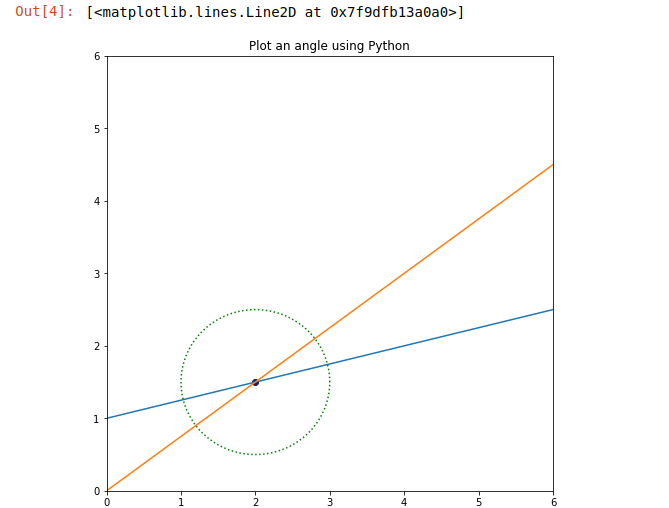4. Mark it as a point where the circle will intersect with straight lines and plot those two points where we find the angle between them

Now, let’s find the points where the circle is intersecting the two straight lines. Read the comments below and understand how to mark points. After that color i.e. “crimson” color is provided where the circle will intersect two straight lines. After that names are provided to the points as Point_P1, Point_P2 where we find the angle between them and marked it as black colored as shown in the output.

## Python3

 `# import packages``import` `matplotlib.pyplot as plt``import` `numpy as np` `# slope  and intercepts``a1, b1 ``=` `(``1``/``4``), ``1.0``a2, b2 ``=` `(``3``/``4``), ``0.0` `# The numpy.linspace() function returns``# number spaces evenly w.r.t interval``l ``=` `np.linspace(``-``6``, ``6``, ``100``)` `# use to create new figure``plt.figure(figsize``=``(``8``, ``8``))` `# plotting``plt.xlim(``0``, ``6``)``plt.ylim(``0``, ``6``)``plt.title(``'Plot an angle using Python'``)``plt.plot(l, l``*``a1``+``b1)``plt.plot(l, l``*``a2``+``b2)` `# intersection point``x0 ``=` `(b2``-``b1)``/``(a1``-``a2)``y0 ``=` `a1``*``x0 ``+` `b1``plt.scatter(x0, y0, color``=``'midnightblue'``)` `# circle for angle``theta ``=` `np.linspace(``0``, ``2``*``np.pi, ``100``)``r ``=` `1.0``x1 ``=` `r ``*` `np.cos(theta) ``+` `x0``x2 ``=` `r ``*` `np.sin(theta) ``+` `y0``plt.plot(x1, x2, color``=``'green'``, linestyle``=``'dotted'``)`  `# intersection points``x_points ``=` `[]``y_points ``=` `[]` `# Code for Intersecting points of circle with Straight Lines``def` `intersection_points(slope, intercept, x0, y0, radius):``    ``a ``=` `1` `+` `slope``*``*``2``    ``b ``=` `-``2.0``*``x0 ``+` `2``*``slope``*``(intercept ``-` `y0)``    ``c ``=` `x0``*``*``2` `+` `(intercept``-``y0)``*``*``2` `-` `radius``*``*``2` `    ``# solving the quadratic equation:``    ``delta ``=` `b``*``*``2` `-` `4.0``*``a``*``c  ``# b^2 - 4ac``    ``x1 ``=` `(``-``b ``+` `np.sqrt(delta)) ``/` `(``2.0` `*` `a)``    ``x2 ``=` `(``-``b ``-` `np.sqrt(delta)) ``/` `(``2.0` `*` `a)` `    ``x_points.append(x1)``    ``x_points.append(x2)` `    ``y1 ``=` `slope``*``x1 ``+` `intercept``    ``y2 ``=` `slope``*``x2 ``+` `intercept` `    ``y_points.append(y1)``    ``y_points.append(y2)` `    ``return` `None`  `# Finding the intersection points for line1 with circle``intersection_points(a1, b1, x0, y0, r)` `# Finding the intersection points for line1 with circle``intersection_points(a2, b2, x0, y0, r)` `# Here we plot Two points in order to find angle between them``plt.scatter(x_points[``0``], y_points[``0``], color``=``'crimson'``)``plt.scatter(x_points[``2``], y_points[``2``], color``=``'crimson'``)`  `# Naming the points.``plt.text(x_points[``0``], y_points[``0``], ``'  Point_P1'``, color``=``'black'``)``plt.text(x_points[``2``], y_points[``2``], ``'  Point_P2'``, color``=``'black'``)`

Output: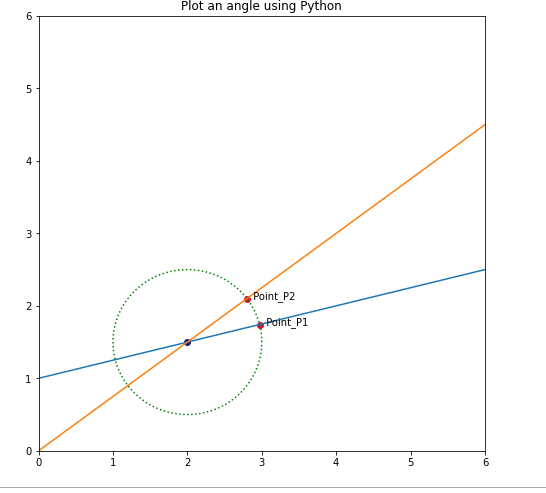5. Calculate the angle and Plot Angle

In the below code the angle between to points Point_P1 and Point_P2 is calculated and finally, it is plotted as shown in the output.

## Python3

 `# import packages``import` `matplotlib.pyplot as plt``import` `numpy as np` `# slope  and intercepts``a1, b1 ``=` `(``1``/``4``), ``1.0``a2, b2 ``=` `(``3``/``4``), ``0.0` `# The numpy.linspace() function returns``# number spaces evenly w.r.t interval``l ``=` `np.linspace(``-``6``, ``6``, ``100``)` `# use to create new figure``plt.figure(figsize``=``(``8``, ``8``))` `# plotting``plt.xlim(``0``, ``6``)``plt.ylim(``0``, ``6``)``plt.title(``'Plot an angle using Python'``)``plt.plot(l, l``*``a1``+``b1)``plt.plot(l, l``*``a2``+``b2)` `# intersection point``x0 ``=` `(b2``-``b1)``/``(a1``-``a2)``y0 ``=` `a1``*``x0 ``+` `b1``plt.scatter(x0, y0, color``=``'midnightblue'``)` `# circle for angle``theta ``=` `np.linspace(``0``, ``2``*``np.pi, ``100``)``r ``=` `1.0``x1 ``=` `r ``*` `np.cos(theta) ``+` `x0``x2 ``=` `r ``*` `np.sin(theta) ``+` `y0``plt.plot(x1, x2, color``=``'green'``, linestyle``=``'dotted'``)`  `# intersection points``x_points ``=` `[]``y_points ``=` `[]` `# Code for Intersecting points of circle with Straight Lines``def` `intersection_points(slope, intercept, x0, y0, radius):``    ``a ``=` `1` `+` `slope``*``*``2``    ``b ``=` `-``2.0``*``x0 ``+` `2``*``slope``*``(intercept ``-` `y0)``    ``c ``=` `x0``*``*``2` `+` `(intercept``-``y0)``*``*``2` `-` `radius``*``*``2` `    ``# solving the quadratic equation:``    ``delta ``=` `b``*``*``2` `-` `4.0``*``a``*``c  ``# b^2 - 4ac``    ``x1 ``=` `(``-``b ``+` `np.sqrt(delta)) ``/` `(``2.0` `*` `a)``    ``x2 ``=` `(``-``b ``-` `np.sqrt(delta)) ``/` `(``2.0` `*` `a)` `    ``x_points.append(x1)``    ``x_points.append(x2)` `    ``y1 ``=` `slope``*``x1 ``+` `intercept``    ``y2 ``=` `slope``*``x2 ``+` `intercept` `    ``y_points.append(y1)``    ``y_points.append(y2)` `    ``return` `None`  `# Finding the intersection points for line1 with circle``intersection_points(a1, b1, x0, y0, r)` `# Finding the intersection points for line1 with circle``intersection_points(a2, b2, x0, y0, r)` `# Here we plot Two ponts in order to find angle between them``plt.scatter(x_points[``0``], y_points[``0``], color``=``'crimson'``)``plt.scatter(x_points[``2``], y_points[``2``], color``=``'crimson'``)`  `# Naming the points.``plt.text(x_points[``0``], y_points[``0``], ``'  Point_P1'``, color``=``'black'``)``plt.text(x_points[``2``], y_points[``2``], ``'  Point_P2'``, color``=``'black'``)`  `# plot angle value` `def` `get_angle(x, y, x0, y0, radius):` `    ``base ``=` `x ``-` `x0``    ``hypotenuse ``=` `radius` `    ``# calculating the angle for a intersection point``    ``# which is equal to the cosine inverse of (base / hypotenuse)``    ``theta ``=` `np.arccos(base ``/` `hypotenuse)` `    ``if` `y``-``y0 < ``0``:``        ``theta ``=` `2``*``np.pi ``-` `theta` `    ``print``(``'theta='``, theta, ``',theta in degree='``, np.rad2deg(theta), ``'\n'``)` `    ``return` `theta`  `theta_list ``=` `[]` `for` `i ``in` `range``(``len``(x_points)):` `    ``x ``=` `x_points[i]``    ``y ``=` `y_points[i]` `    ``print``(``'intersection point p{}'``.``format``(i))``    ``theta_list.append(get_angle(x, y, x0, y0, r))` `    ``# angle for intersection point1 ( here point p1 is taken)``p1 ``=` `theta_list[``0``]` `# angle for intersection point2 ( here point p4 is taken)``p2 ``=` `theta_list[``2``]` `# all the angles between the two intersection points``theta ``=` `np.linspace(p1, p2, ``100``)` `# calculate the x and y points for``# each angle between the two intersection points``x1 ``=` `r ``*` `np.cos(theta) ``+` `x0``x2 ``=` `r ``*` `np.sin(theta) ``+` `y0` `# plot the angle``plt.plot(x1, x2, color``=``'black'``)` `# Code to print the angle at the midpoint of the arc.``mid_angle ``=` `(p1 ``+` `p2) ``/` `2.0` `x_mid_angle ``=` `(r``-``0.5``) ``*` `np.cos(mid_angle) ``+` `x0``y_mid_angle ``=` `(r``-``0.5``) ``*` `np.sin(mid_angle) ``+` `y0` `angle_in_degree ``=` `round``(np.rad2deg(``abs``(p1``-``p2)), ``1``)` `plt.text(x_mid_angle, y_mid_angle, angle_in_degree, fontsize``=``12``)` `# plotting the intersection points``plt.scatter(x_points[``0``], y_points[``0``], color``=``'red'``)``plt.scatter(x_points[``2``], y_points[``2``], color``=``'red'``)``plt.show()`

Output: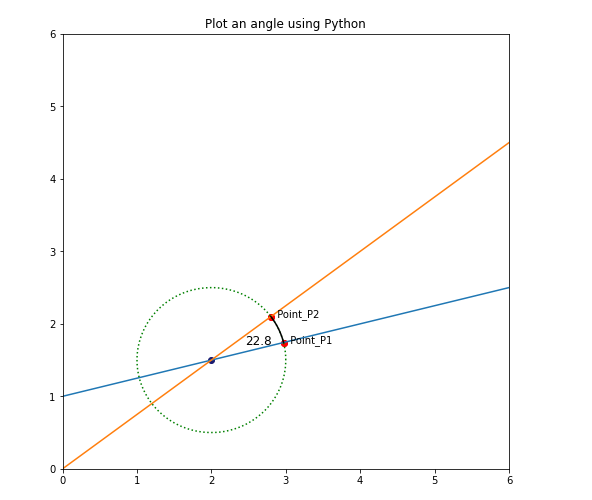Attention geek! Strengthen your foundations with the Python Programming Foundation Course and learn the basics.

To begin with, your interview preparations Enhance your Data Structures concepts with the Python DS Course.

My Personal Notes arrow_drop_up